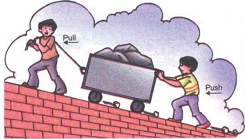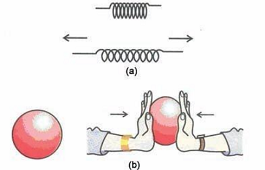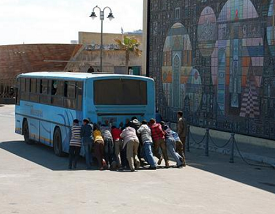# what is Force

## Force and pressure of Class 8

Force is that agency which can change the state of rest or of uniform motion or shape or direction of motion of anybody. The unit of force in M.K.S. system is Newton.

Consider a ball kept on a table, we can move it by pulling or pushing. We can increase its speed by pushing it in the direction of motion, if we push it opposite to the direction of motion its speed will decrease. If the ball is in motion towards east, we push it towards north the direction will change. Take a soft rubber ball between your palms and push the ball from both sides the shape of the ball will change. In all these cases we have applied the force on the ball.

So force is a push or pull which can move the object. It can change the speed of the object, it can change the direction of motion. It can change the shape of the object. In all the above cases we have applied the force on the ball and the ball is accelerated so we can define force as follows.

"Force is the cause which can produce acceleration in the body on which it acts".

### EFFECTS OF FORCE:

Force is that physical quantity that changes or tends to change the state of rest or uniform motion of an object in a straight line.

• Force can make a stationary object move and can change the speed of the object:
• e.g.
• (A)   A stationary football can be made to move by giving it a small push.
• (B)   The moving toy car can be made to stop by applying a force.
• (C)   A tractor can move a trolley by pulling it.
• (D)   We push door to open or close it.• ### Force can change the direction of a moving object:

When a force is applied on a moving object perpendicular to its motion, it changes the direction of the motion of the object. It can also change the velocity of the object.

e.g.
• (A)   A cricketer applies a tangential force to change the direction of the cricket ball.
• (B)   When a football in motion kicked perpendicular to its motion, direction of motion changes.
• (C)   When we hit a cricket ball with a bat, the direction in which the ball is moving changes.To score More in your class 8 refer NCERT solutions for class 8 .

A force applied on an object can also change its shape and size.### MATHEMATICAL REPRESENTATION OF FORCE:

Mathematically, force F is equal to the product of mass m of a body and acceleration a. produced in the body due to that force.

i.e.   F = ma

• Force can change the shape and size of the object:

• e.g.
• (A)   When we compress a spring then its length decreases.
• (B)   When we stress a spring then its length increases.
• (C)   When we apply force on a balloon then its shape changes.
• (D)   Force can stop the moving objects or slow them down:

On applying brakes to a moving bicycle, it first slows down and then stops. A cricket ball is stopped by a player by applying a force in the direction opposite to that of the ball. Imagine your friend is riding a bicycle. If you pull his bicycle from behind it is going to slow down. If ball is thrown vertically upwards, the pull of the earth causes the speed of the ball to decrease. It means that if you apply a force in the direction opposite to the direction of the moving body, the body slows down.

• (E)   A vehicle can be made to stop by applying brakes on it.